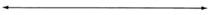# 6.5: Factoring by Grouping

$$\newcommand{\vecs}{\overset { \rightharpoonup} {\mathbf{#1}} }$$ $$\newcommand{\vecd}{\overset{-\!-\!\rightharpoonup}{\vphantom{a}\smash {#1}}}$$$$\newcommand{\id}{\mathrm{id}}$$ $$\newcommand{\Span}{\mathrm{span}}$$ $$\newcommand{\kernel}{\mathrm{null}\,}$$ $$\newcommand{\range}{\mathrm{range}\,}$$ $$\newcommand{\RealPart}{\mathrm{Re}}$$ $$\newcommand{\ImaginaryPart}{\mathrm{Im}}$$ $$\newcommand{\Argument}{\mathrm{Arg}}$$ $$\newcommand{\norm}{\| #1 \|}$$ $$\newcommand{\inner}{\langle #1, #2 \rangle}$$ $$\newcommand{\Span}{\mathrm{span}}$$ $$\newcommand{\id}{\mathrm{id}}$$ $$\newcommand{\Span}{\mathrm{span}}$$ $$\newcommand{\kernel}{\mathrm{null}\,}$$ $$\newcommand{\range}{\mathrm{range}\,}$$ $$\newcommand{\RealPart}{\mathrm{Re}}$$ $$\newcommand{\ImaginaryPart}{\mathrm{Im}}$$ $$\newcommand{\Argument}{\mathrm{Arg}}$$ $$\newcommand{\norm}{\| #1 \|}$$ $$\newcommand{\inner}{\langle #1, #2 \rangle}$$ $$\newcommand{\Span}{\mathrm{span}}$$$$\newcommand{\AA}{\unicode[.8,0]{x212B}}$$

## Using Grouping to Factor a Polynomial

Sometimes a polynomial will not have a particular factor common to every term. However, we may still be able to produce a factored form for the polynomial.

The polynomial $$x^3+3x^2−6x−18$$ has no single factor that is common to every term. However, we notice that if we group together the first two terms and the second two terms, we see that each resulting binomial has a particular factor common to both terms.Factor $$x^2$$ out of the first two terms, and factor $$-6$$ out of the second two terms.

$$x^2(x+3) - 6(x+3)$$

Now look closely at the binomial. Each of the two terms contains the factor $$x+3$$.

Factor out $$(x+3)$$.

$$(x+3)(x^2-6)$$ is the final factorization

$$x^3+3x^2−6x−18 = (x+3)(x^2-6)$$

## Knowing when to Try the Grouping Method

We are alerted to the idea of grouping when the polynomial we are considering has either of these qualities:

1. no factor common to all terms
2. an even number of terms

When factoring by grouping, the sign ($$+$$ or $$−$$) of the factor we are taking out will usually (but not always) be the same as the sign of the first term in that group.

## Sample Set A

##### Example $$\PageIndex{1}$$

Factor $$8a^2b^4 - 4b^4 + 14a^2 - 7$$

1. We notice there is no factor common to all terms.
2. We see there are four terms, an even number.
3. We see that terms 1 and 2 have $$+4b^4$$ in common (since the 1st term in the group is $$+8a^2b^4$$).
4. We notice that the 3rd and 4th terms have $$+7$$ in common (since the 1st term in the group is $$+14a^2$$).$$8a^2b^4-4b^4+14a^2-7 = (2a^2-1)(4b^4+7)$$

## Practice Set A

Use the grouping method to factor the following polynomials.

##### Practice Problem $$\PageIndex{1}$$

$$ax+ay+bx+by$$

$$(a+b) (x+y)$$

##### Practice Problem $$\PageIndex{2}$$

$$2am+8m+5an+20n$$

$$(2m+5n) (a+4)$$

##### Practice Problem $$\PageIndex{3}$$

$$a^2x^3 + 4a^2y^3 + 3bx^3 + 12by^3$$

$$(a^2+3b)(x^3 + 4y^3)$$

##### Practice Problem $$\PageIndex{4}$$

$$15mx+10nx−6my−4ny$$

$$(5x−2y) (3m+2n)$$

##### Practice Problem $$\PageIndex{5}$$

$$40abx - 24abxy - 35c^2x + 21c^2xy$$

$$x(8ab−7c^2) (5−3y)$$

##### Practice Problem $$\PageIndex{6}$$

When factoring the polynomial $$8a^2b^4−4b^4+14a^2−78$$ in Sample Set A, we grouped together terms 1 and 2 and 3 and 4. Could we have grouped together terms1 and 3 and 2 and 4? Try this.

$$8a^2b^4−4b^4+14a^2−78 =$$

Yes

Do we get the same result? If the results do not look precisely the same, recall the commutative property of multiplication.

## Exercises

For the following problems, use the grouping method to factor the polynomials. Some polynomials may not be factorable using the grouping method.

##### Exercise $$\PageIndex{1}$$

$$2ab+3a+18b+27$$

$$(2b+3)(a+9)$$

##### Exercise $$\PageIndex{2}$$

$$xy−7x+4y−28$$

##### Exercise $$\PageIndex{3}$$

$$xy+x+3y+3$$

$$(y+1)(x+3)$$

##### Exercise $$\PageIndex{4}$$

$$mp+3mq+np+3nq$$

##### Exercise $$\PageIndex{5}$$

$$ar+4as+5br+20bs$$

$$(a+5b)(r+4s)$$

##### Exercise $$\PageIndex{6}$$

$$14ax−6bx+21ay−9by$$

##### Exercise $$\PageIndex{7}$$

$$12mx−6bx+21ay−9by$$

$$3(4mx−2bx+7ay−3by)$$ Not factorable by grouping

##### Exercise $$\PageIndex{8}$$

$$36ak−8ah−27bk+6bh$$

##### Exercise $$\PageIndex{9}$$

$$a^2b^2 + 2a^2 + 3b^2 + 6$$

$$(a^2+3)(b^2+2)$$

##### Exercise $$\PageIndex{10}$$

$$3n^2 + 6n + 9m^3 + 12m$$

##### Exercise $$\PageIndex{11}$$

$$8y^4 - 5y^3 + 12z^2 - 10z$$

Not factorable by grouping

##### Exercise $$\PageIndex{12}$$

$$x^2 + 4x - 3y^2 + y$$

##### Exercise $$\PageIndex{13}$$

$$x^2 - 3x + xy - 3y$$

$$(x+y)(x−3)$$

##### Exercise $$\PageIndex{14}$$

$$2n^2+12n−5mn−30m$$

##### Exercise $$\PageIndex{15}$$

$$4pq−7p+3q^2−21$$

Not factorable by grouping

##### Exercise $$\PageIndex{16}$$

$$8x^2+16xy−5x−10y$$

##### Exercise $$\PageIndex{17}$$

$$12s^2−27s−8st+18t$$

$$(4s−9)(3s−2t)$$

##### Exercise $$\PageIndex{18}$$

$$15x^2−12x−10xy+8y$$

##### Exercise $$\PageIndex{19}$$

$$a^4b^4+3a^5b^5+2a^2b^2+6a^3b^3$$

$$a^2b^2(a^2b^2 + 2)(1 + 3ab)$$

##### Exercise $$\PageIndex{20}$$

$$4a^3bc−14a^2bc^3+10abc^2−35bc^4$$

##### Exercise $$\PageIndex{21}$$

$$5x^2y^3z+3x^3yw−10y^3z^2−6wxyz$$

$$y(5y^2z+3xw)(x^2−2z)$$

##### Exercise $$\PageIndex{22}$$

$$a^3b^2cd+abc^2dx−a^2bxy−cx^2y$$

##### Exercise $$\PageIndex{23}$$

$$5m^{10}n^{17}p^3 - m^6n^7p^4 - 40m^4n^{10}qt^2 + 8pqt^2$$

$$(m^6n^7p^3−8qt^2)(5m^4n^{10}−p)$$

## Exercises for Review

##### Exercise $$\PageIndex{24}$$

Simplify $$(x^5y^3)(x^2y)$$

##### Exercise $$\PageIndex{25}$$

Use scientific notation to find the product of $$(3 \times 10^{-5})(2 \times 10^2)$$.

$$6 \times 10^{-3}$$

##### Exercise $$\PageIndex{26}$$

Find the domain of the equation $$y = \dfrac{6}{x+5}$$

##### Exercise $$\PageIndex{27}$$

Construct the graph of the inequality $$y \ge -2$$##### Exercise $$\PageIndex{28}$$
Factor $$8a^4b^4 + 12a^3b^5 - 8a^2b^3$$